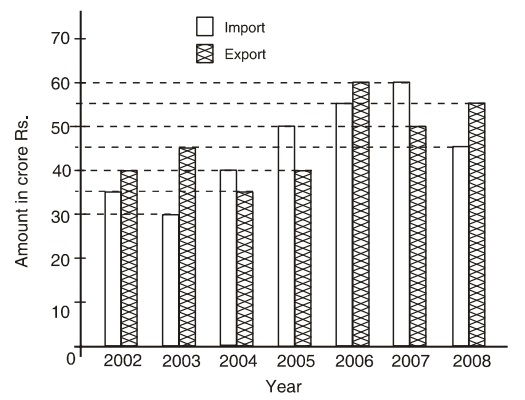Home » Data Interpretation » Bar graph » Question

#### Data Interpretation

Direction: Study the following graph carefully to answer the questions given below it.
Imports and Exports of spare parts by an automobile company over the given years.1. During which year the percentage rise/fall in imports from the previous year is the lowest?
1. 2003
2. 2004
3. 2006
4. 2007
##### Correct Option: D

As per given graph, we calculate the difference in two consecutive years import.
In 2003, change in imports = (35 - 30)/35 x 100 = 100/7 = 139/7% fall
In In 2004, change in imports = (40 - 30)/ 50 x 100 = 1/3 x 100 = 331/3% rise
In 2006, change in imports = (55 - 50)/50 x 100 = 10% rise
In 2007, change in imports = (60 - 55/55) x 100 = 91/11% rise
Hence, in 2007 the percentage rise/fall in imports from the previous year is the lowest.